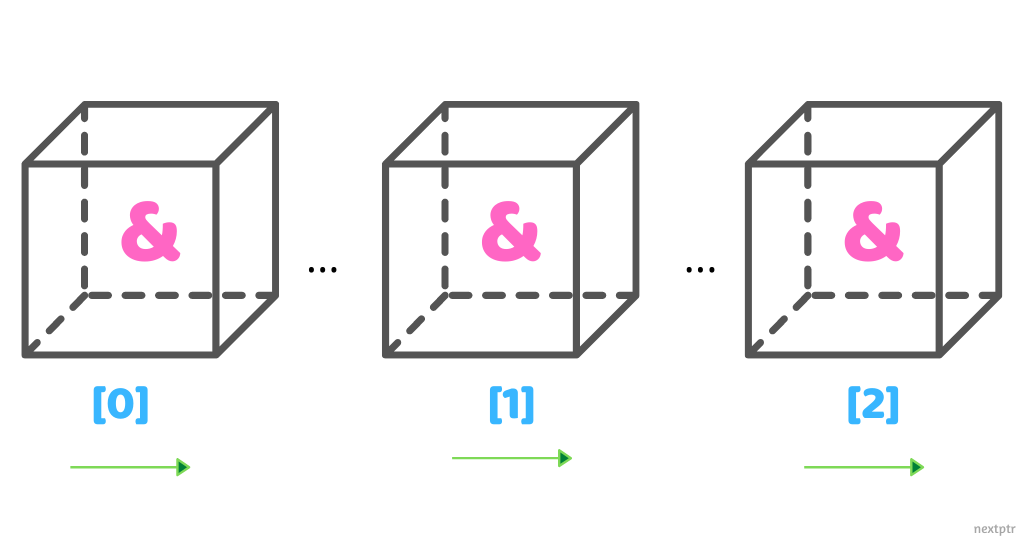# Array of reference_wrapper: an alternate array of references## Overview

A reference is an alias of an object that must be initialized with a valid object before it can be used. References are non-nullable and capture the by-reference intent better than pointers.

However, because of their nature, references have some pain points associated with them. For one, it is not possible to have an array of references. The reason why an array of references is illegal is not that references must be initialized before they can be used. After all, the following form does initialize all the array elements, but it is still invalid:

``````int a=0,b=0,c=0;
int& arr[] = {a, b, c}; //Error
``````

An array of references is illegal because a reference is not an object. According to the C++ standard, an object is a region of storage, and it is not specified if a reference needs storage (Standard §11.3.2/4).

Thus, `sizeof` does not return the size of a reference, but the size of the referred object. We cannot declare a pointer or a reference to a reference. Compilers are free to optimize away the references to local objects. The C++ standard explicitly states that:

There shall be no references to references, no arrays of references, and no pointers to references (§11.3.2/5).

## Array of reference_wrapper as an alternate

If we need an array of object references, we don't have to resort to the workaround of storing pointers in an array and give up the non-nullable safety of references. The standard library provides std::reference_wrapper<T>.

A reference_wrapper<T> is an object that wraps a pointer (T*) and acts as a reference (T&). It is assignable and copyable, and therefore, it can rebind to another object like a pointer:

``````std::string str1{"Hello"};
std::string str2{"World"};

//std::ref creates a reference_wrapper
auto r1 = std::ref(str1); //OK
auto r2 = std::ref(str2); //OK

std::cout << r1.get() << " " << r2.get(); //Hello World

//Assignment rebinds the reference_wrapper
r2 = r1;  //r2 also refers to str1 now

//Implicit conversion to std::string&
std::string cstr = r2; //cstr is "Hello"
``````

So, it is possible to create an array of reference_wrapper. However, like a reference, a reference_wrapper cannot be null. Therefore, it cannot be default constructed and must be initialized with a valid object. Consequently, we cannot create an uninitialized array of reference_wrapper. All elements of a reference_wrapper array must be initialized, as follows:

``````//Possible to create an array of reference_wrapper
std::string hello{"Hello"};
std::string world{"World"};
std::reference_wrapper<std::string> arr[] = {hello, world}; //OK

std::cout << arr.get() << " " << arr.get(); //Hello World
``````

## Concept Check

So, we cannot create an array of references, but it is possible to create an array of reference_wrapper as an alternative. But what if we need to create an array of class that has a reference or reference_wrapper member? Consider the following two classes — BoxRef and BoxRefWrap:

``````struct BoxRef {
BoxRef(int& r):ref(r){}
int& ref;
};

struct BoxRefWrap {
BoxRefWrap(int& r):ref(r){}
std::reference_wrapper<int> ref;
};
``````

We declare and initialize two arrays, as follows:

``````int m=10, n=20;

BoxRefWrap boxRefWraps[] = {m, n};

BoxRef boxRefs[] = {m, n};
``````

Which array declaration(s) from above is/are legal (would compile)? Select the answer below and check the `Explanations` for details:

## Afterword

An array of reference_wrapper must be initialized at declaration. If we need the flexibility of the uninitialized collection of reference_wrapper, then consider a vector. Unlike an array, a vector grows on demand. Therefore, it is possible to create an empty vector of reference_wrapper, to which the elements can be added when required:

``````std::vector<std::reference_wrapper<int>> v; //OK

int z = 10;
v.push_back(std::ref(z)); //OK
``````